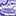# New to Qlik Sense

If you’re new to Qlik Sense, start with this Discussion Board and get up-to-speed quickly.

Announcements
Qlik Insider: SaaS capabilities for Data Integration and Analytics, May 25th! Register Now
cancel
Showing results for
Did you mean:Creator II

## Percentage calculation Help

Hi Experts,

I have Two KPIs Current Month Amount and Previous Month Amount

[CM Amount] =Count({<[Month Year]={\$(=Max([Month Year]))}>}Sales)          Result : 32.68

[PM Amount] =Count({<[Month Year]={\$(=Max([Month Year])-1)}>}Sales)      Result : 27.31

Please help me to write the expression for how much Amount Percentage increased in Current Month compared to Previous Month.

1 Solution

Accepted SolutionsPartner

you can try this

(

Count({<[Month Year]={\$(=Max([Month Year]))}>}Sales)

/

Count({<[Month Year]={\$(=Max([Month Year])-1)}>}Sales)

)

-1

the increase would be 20%

so you can get the percentage increase2 RepliesCreator II

Hi,

Calculating Percentage ChangePartner

you can try this

(

Count({<[Month Year]={\$(=Max([Month Year]))}>}Sales)

/

Count({<[Month Year]={\$(=Max([Month Year])-1)}>}Sales)

)

-1

the increase would be 20%

so you can get the percentage increaseTags